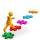Triplets

Three years ago, the triplets Pavel, Petr, and Luke and their five-year-old sister Jane had together 25 years. How old is Jane today?

Result

j =  13

Solution:

(j-3) + 3(j-3-5) = 25

4j = 52

j = 13

Calculated by our simple equation calculator.

Leave us a comment of example and its solution (i.e. if it is still somewhat unclear...):Be the first to comment!To solve this verbal math problem are needed these knowledge from mathematics:

Do you have a linear equation or system of equations and looking for its solution? Or do you have quadratic equation?

Next similar examples:

1. Three brothersThe three brothers have a total of 42 years. Jan is five years younger than Peter and Peter is 2 years younger than Michael. How many years has each of them?
2. Father and sonWhen I was 11, my father was 35 years old. Today, my father has three times more years than me. How old is she?
3. Father and daughterWhen I was 11 my father was 35 year old. Now, father has three times older then me. How old is she?
4. SchoolsThree schools are attended by 678 pupils. To the first attend 21 students more and to the third 108 fewer students than to second school. How many students attend the schools?
5. Family parcelsIn father will he divided the land so that the older son had three bigger part than younger son. Later elder son gave 2.5 ha field to younger and they had both the same. Determine the area of family parcel.
6. Journey to schoolOn the way to school I went steady step. In the first half of the journey, I counted every second step, in the second half every third. How many steps I do when I went to school if I counted double step are 250 more than three steps?
7. Three figures - numbersThe sum of three numbers, if each is 10% larger than the previous one, is 662. Determine the figures.
8. Six yearsIn six years Jan will be twice as old as he was six years ago. How old is he?
9. ATC campThe owner of the campsite offers 79 places in 22 cabins. How many of them are triple and quadruple?
10. BlackberriesDaniel, Jolana and Stano collected together 34 blackberries. Daniel collected 8 blackberries more than Jolana, Jolana 4 more than Stano. Determine the number blackberries each collected .
11. Belgium vs ItalyBelgium played a match with Italy and Belgium win by 2 goals. The match fell a total 6 goals. Determine the number of goals scored by Belgium and by Italy.
12. TreesAlong the road were planted 250 trees of two types. Cherry for 60 CZK apiece and apple 50 CZK apiece. The entire plantation cost 12,800 CZK. How many was cherries and apples?
13. Linear systemSolve this linear system (two linear equations with two unknowns): x+y =36 19x+22y=720
14. Football seasonDalibor and Adam together scored 97 goals in the season. Adam scored 9 goals more than Dalibor. How many goals scored each?
15. 13 ticketsA short and long sightseeing tour is possible at the castle. Ticket for a short sightseeing circuit costs CZK 60, for a long touring circuit costs CZK 100. So far, 13 tickets have been sold for 1140 CZK. How much did you pay for tickets for a short tour?
16. Fifth of the numberThe fifth of the number is by 24 less than that number. What is the number?
17. Equations - simpleSolve system of linear equations: x-2y=6 3x+2y=4